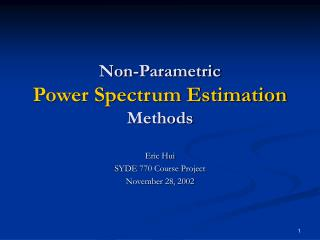Download PresentationNon-Parametric Power Spectrum Estimation Methods

# Non-Parametric Power Spectrum Estimation Methods - PowerPoint PPT PresentationDownload Presentation## Non-Parametric Power Spectrum Estimation Methods

- - - - - - - - - - - - - - - - - - - - - - - - - - - E N D - - - - - - - - - - - - - - - - - - - - - - - - - - -
##### Presentation Transcript

1. Non-ParametricPower Spectrum Estimation Methods Eric Hui SYDE 770 Course Project November 28, 2002

2. Introduction • Applications of Power Spectrum Estimation (PSE): • Wiener Filter • Feature Extraction • Non-parametric PSE does NOT assume any data-generating process or model (e.g. autoregressive model).

3. Motivation • Ideal autocorrelation: • Actual autocorrelation: • Limited (finite length of) data due to: • Availability of data • Assumption of stationary

4. x(n) xN(n) n n 0 0 N N Periodogram Method redefined as DTFT

5. k k Periodogram Method -N 0 N DTFT DTFT 0

6. PSD PSD k k PSD PSD k “Good” Method? • Necessary conditions for mean-square convergence: • Asymptotically Unbiased • Zero Variance as N ↑ as N ↑ k

7. PSD PSD PSD as N ↑ k k k PSD PSD as N ↑ k k Evaluation of Methods • Resolution • How much “blurring” effect is there on the power spectrum? • Bias (Asymptotic) • Does the estimation approach the true value with more data (i.e. as N increases)? • Variance • Does the amount of deviation from the true value depend on the data length (i.e. N)?

8. k -N 0 N DTFT PSD PSD as N ↑ k k k 0 Different PSE Methods • Periodogram Method • Apply rectangular window to x(n) to get xN(n). • Modified Periodogram Method • Apply non-rectangular window to x(n) to get xN(n). • Bartlett’s Method • Average the Periodogram estimate of non-overlapping sub-intervals of x(n). • Welch’s Method • Average the Modified Periodogram estimate of overlappingsub-intervals of x(n). • Blackman-Turkey Method • Apply non-triangular window to r(x).

9. Application: Feature Extraction PSD linearize repeat for whole image